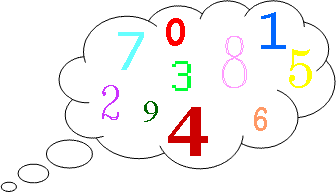#### You may also like### Consecutive Numbers

An investigation involving adding and subtracting sets of consecutive numbers. Lots to find out, lots to explore.### Calendar Capers

Choose any three by three square of dates on a calendar page...### Days and Dates

Investigate how you can work out what day of the week your birthday will be on next year, and the year after...

# Think of Two Numbers

##### Age 11 to 14Challenge Level

Here is an alternative and more unusual version of the "Think of a Number" trick which you may have heard of before.Think of two whole numbers under $10$.

Take one of them and add $1$.
Multiply by $5$.
Add $1$ again.
Double your answer.
Subtract $1$.

Add your second number.
Add $2$.
Double again.
Subtract $8$.
Halve this number and tell me your answer.

From your answer I can work out both your numbers very quickly. How?

Choose some different pairs of numbers and repeat the process.
Can you figure out how the trick works?